# Exponential-time algorithm

## Algorithm for graph homomorphisms ★★

Author(s): Fomin; Heggernes; Kratsch

Question

Is there an algorithm that decides, for input graphsand, whether there exists a homomorphism fromtoin time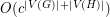for some constant?

## Exponential Algorithms for Knapsack ★★

Author(s): Lipton

Conjecture

The famous 0-1 Knapsack problem is: Given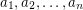andintegers, determine whether or not there arevaluesso that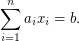The best known worst-case algorithm runs in time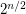times a polynomial in. Is there an algorithm that runs in time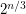?